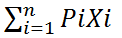# Algorithm for fractional knapsack problem

Submitted by Abhishek Kataria, on August 02, 2018

## Knapsack problem

The knapsack problem or rucksack problem is a problem in combinative or integrative optimization. In this kind of problem, there are set of items are given with a weight and a value, determine the number of each item included in a collection so that the total weight is less than or equal to the given limit and the total value is as large as possible.

## Fractional Knapsack problem

In fractional knapsack fractions of an item can be taken rather than having to make a binary choice for each of them. In this objective function is mathematically represented by:

MaxWhere, Pi= profit and Xi = fraction.Where, Wi = weight of the corresponding and Xi= fraction.

In this, at last, the output should be an array of the fraction item that we have taken, and in this, we also have to take output that gives the maximum profit.

### Algorithm for fractional knapsack

```1.	W and item have value Vi and weight Wi.
2.	Rank item by value/weight ratio: Vi/Wi.
3.	Thus : Vi/Wi= Vj/Wj for all i<=Wj.
4.	Consider items in order of descending ratio.
5.	Take as much of each item is possible.
6.	Assume value and weight arrays are
sorted by Vi<=Wi fractional knapsack (V,w,W)
8.	i:=1
9.	while load<w and i<=n loop
10.	 if
11.	 wi <=W –loop then
12.	  take all of item i
13.	    else
14.	           take (W-load)/wi of item i
15.	   end if
17.	 i:i+1
18.	end loop
19.	return loop
```

Example of fractional knapsack for the following instance by using greedy approach in which maximum value, M =25kg.

S.no Weight Profit
1 10 30
2 5 20
3 15 40
4 8 36

P=

 30 20 40 36

W=

 10 5 15 8

Now we will calculate the profit per unit capacity of knapsack: P/W

 3 4 2.6 4.5

Now the fraction i.e. X

 1 1 2/15 1

i.e. to calculate a total profit:

```P   = P1X1 + P2X2 + P3X3 + P4X4
= 30×1+20×1+40×2/15+36×1
= 91.33
```

Preparation

What's New

Top Interview Coding Problems/Challenges!